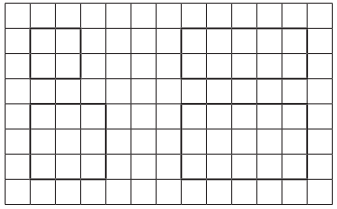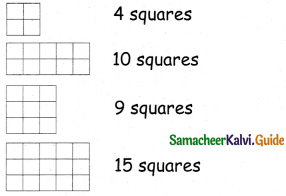Students can download 5th Maths Term 3 Chapter 1 Geometry InText Questions and Answers, Notes, Samacheer Kalvi 5th Maths Guide Pdf helps you to revise the complete Tamilnadu State Board New Syllabus, helps students complete homework assignments and to score high marks in board exams.

## Tamilnadu Samacheer Kalvi 5th Maths Solutions Term 3 Chapter 1 Geometry InText Questions

Activity 1 (Text Book Page No. 2)

Write the perimeter of each figure:
Question 1.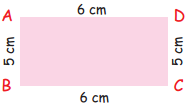5 + 6 + 5 + 6 = 22 cmQuestion 2.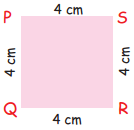4 + 4 + 4 + 4 = 16 cm

Question 3.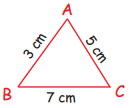3 + 5 + 7 = 15 cmQuestion 4.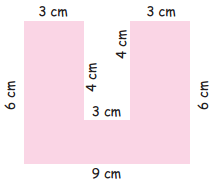6 + 3 + 4 + 3 + 4 + 3 + 6 + 9 = 38 cm

Question 5.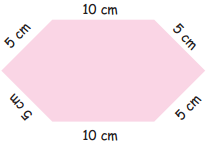5 + 5 + 10 + 5 + 5 + 10 = 40 cmQuestion 6.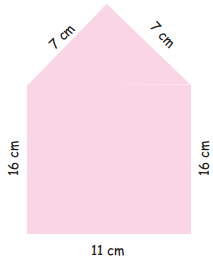11 + 6 + 7 + 7 + 16 = 57 cm

Try This (Text Book Page No. 5)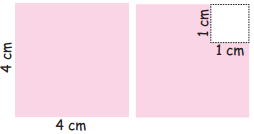If a square of side 1 cm is cut out of the corner of a larger square with side 4 cm (See the figure). What will be the perimeter of the remaining shape?